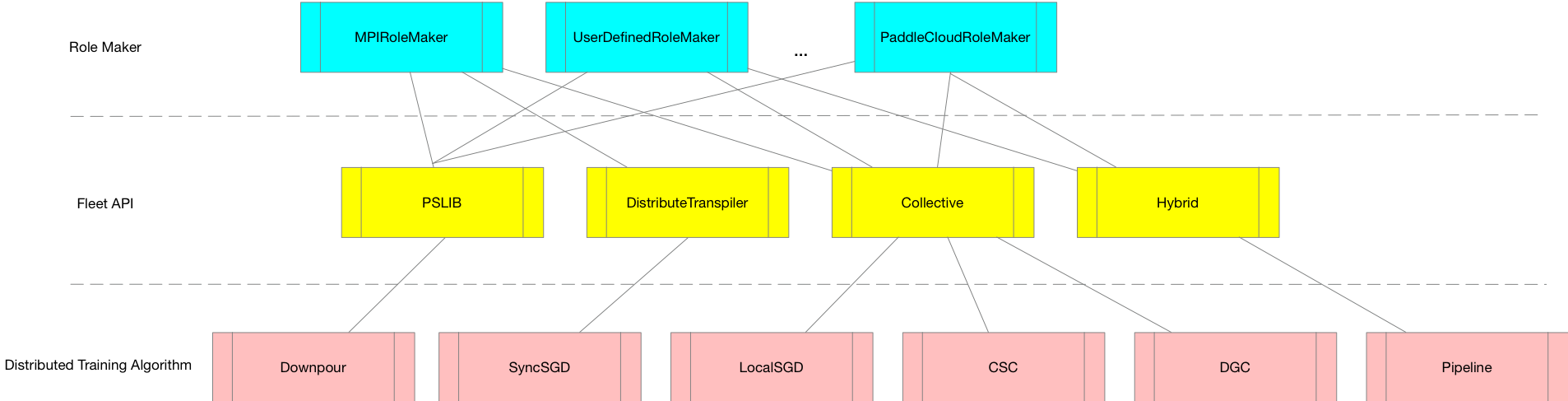# SunGaofeng / Fleet

Distributed Training API and Benchmark on Paddle Fluid

Geek Repo

Github PK Tool

# Fleet

Fleet is High-Level API for distributed training in PaddlePaddle. The name of Fleet means that a large crowd of ships working together to finish a large scale job. The design of Fleet makes a trade-off between easy-to-use and algorithmic extensibility. First, a user can shift from single machine paddle fluid code to distributed code within ten lines of code. Second, different algorithms can be easily defined through distributed strategy through Fleet API.

## Quick Start

Note: all the examples here should be replicated from develop branch of Paddle

We show quick-start examples for user to use Collective training with Fleet API. Multiple GPU training is frequently used in modern AI models that require high performance computing ability of deep learning framework. Sample codes can be found in examples/quick-start folder.

Suppose we define a simple neural nets in examples/quick-start/nets.py

```import paddle.fluid as fluid

def mlp(input_x, input_y, hid_dim=128, label_dim=2):
fc_1 = fluid.layers.fc(input=input_x, size=hid_dim, act='tanh')
fc_2 = fluid.layers.fc(input=fc_1, size=hid_dim, act='tanh')
prediction = fluid.layers.fc(input=[fc_2], size=label_dim, act='softmax')
cost = fluid.layers.cross_entropy(input=prediction, label=input_y)
avg_cost = fluid.layers.mean(x=cost)
return avg_cost```

Simple local training can be defined as in examples/quick-start/local_trainer.py

```import paddle.fluid as fluid
from nets import mlp
from utils import gen_data

input_x = fluid.layers.data(name="x", shape=, dtype='float32')
input_y = fluid.layers.data(name="y", shape=, dtype='int64')

cost = mlp(input_x, input_y)
optimizer = fluid.optimizer.SGD(learning_rate=0.01)
optimizer.minimize(cost)
place = fluid.CUDAPlace(0)

exe = fluid.Executor(place)
exe.run(fluid.default_startup_program())
step = 1001
for i in range(step):
cost_val = exe.run(feed=gen_data(), fetch_list=[cost.name])
print("step%d cost=%f" % (i, cost_val))```

Parameter server training is suitable for parallel training of large-scale data. Based on the definition of single-machine model, we give an example of training with parameter server, source code of this example is in examples/quick-start/distributed_train.py

```import paddle.fluid as fluid
from nets import mlp
from utils import gen_data

input_x = fluid.layers.data(name="x", shape=, dtype='float32')
input_y = fluid.layers.data(name="y", shape=, dtype='int64')

cost = mlp(input_x, input_y)
optimizer = fluid.optimizer.SGD(learning_rate=0.01)

fleet.init(role)
optimizer = fleet.distributed_optimizer(optimizer)
optimizer.minimize(cost)

if fleet.is_server():
fleet.init_server()
fleet.run_server()
elif fleet.is_worker():
place = fluid.CPUPlace()
exe = fluid.Executor(place)
exe.run(fluid.default_startup_program())
step = 1001
for i in range(step):
cost_val = exe.run(
program=fluid.default_main_program(),
feed=gen_data(),
fetch_list=[cost.name])
print("worker_index: %d, step%d cost = %f" %
(fleet.worker_index(), i, cost_val))```

Command for parameter server training with multiple process on CPU is as follows:

``````python -m paddle.distributed.launch_ps distributed_train.py
``````

If you want to use high performance chip to do distributed training, such as distributed GPU training, Fleet API will help you by adding less than 10 lines of code, source code of this example is in examples/quick-start/collective_train.py

```import paddle.fluid as fluid
from nets import mlp
from utils import gen_data

input_x = fluid.layers.data(name="x", shape=, dtype='float32')
input_y = fluid.layers.data(name="y", shape=, dtype='int64')

cost = mlp(input_x, input_y)
optimizer = fluid.optimizer.SGD(learning_rate=0.01)
fleet.init(role)

optimizer = fleet.distributed_optimizer(optimizer)
optimizer.minimize(cost)
place = fluid.CUDAPlace(0)

exe = fluid.Executor(place)
exe.run(fluid.default_startup_program())
step = 1001
for i in range(step):
cost_val = exe.run(
program=fluid.default_main_program(),
feed=gen_data(),
fetch_list=[cost.name])
print("worker_index: %d, step%d cost = %f" %
(fleet.worker_index(), i, cost_val))```

Command for distributed training with multiple process on multiple GPU card is as follows:

``````python -m paddle.distributed.launch collective_train.py
``````

## Design of FleetFleet API aims to help users run distributed training of deep learning models with easy-to-use API. Also, Fleet API maintains the ability to plugin new distributed algorithm by developers of PaddlePaddle.

### Fleet API functions

• init(role_maker=None)

It is responsible for fleet initialization, which needs to be called before using other Fleet interfaces. Basically it is used to define multi-machine environment configuration.

• is_worker()

It is used to check whether the current node is a worker node in parameter server training. If the current node is a worker node,the function will return True, otherwise the function will return False.

• is_server(model_dir=None)

It is used to check whether the current node is a server node in parameter server training. If the current node is a server node,the function will return True, otherwise the function will return False.

• init_server()

Fleet loads model-related parameters stored in model_dir to initialize parameter server in parameter server training.

• run_server()

It is used to start the server service in parameter server training.

• init_worker()

It is used to start the worker service in parameter server training.

• stop_worker()

It is used to terminate the worker service after the parameter server training is done.

• distributed_optimizer(optimizer, strategy=None)

It is used to decorate the distributed optimization algorithm. Users can bring in single optimizer and configure distributed training strategy, the function will return a distributed optimizer

### Role Maker

• MPISymetricRoleMaker

description：MPISymetric RoleMaker assumes that each node starts two processes, one for worker service and one for parameter server service, which requires an MPI environment on the user's cluster.

example

```from paddle.fluid.incubate.fleet.parameter_server.distribute_transpiler import fleet

role = role_maker.MPISymetricRoleMaker()
fleet.init(role)```

command for running the example

``````mpirun -np 2 python trainer.py
``````

Parameter Server training example

```from paddle.fluid.incubate.fleet.parameter_server.distribute_transpiler import fleet

fleet.init(role)```

command for running the example

``````python -m paddle.distributed.launch_ps --worker_num 2 --server_num 2 trainer.py
``````

Collective training example

```from paddle.fluid.incubate.fleet.collective import fleet

fleet.init(role)```

command for running the example

``````python -m paddle.distributed.launch trainer.py
``````
• UserDefinedRoleMaker

description：User-defined node role information, IP and port information.

example

```from paddle.fluid.incubate.fleet.parameter_server.distribute_transpiler import fleet

role = role_maker.UserDefinedRoleMaker(
current_id=int(os.getenv("CURRENT_ID")),
role=role_maker.Role.WORKER if bool(int(os.getenv("IS_WORKER")))
else role_maker.Role.SERVER,
worker_num=int(os.getenv("WORKER_NUM")),
server_endpoints=pserver_endpoints)
fleet.init(role)```

### Strategy

Parameter Server Training

• Sync_mode

Collective Training

• LocalSGD

### Fleet Mode

#### Parameter Server Training

`from paddle.fluid.incubate.fleet.parameter_server.distribute_transpiler import fleet`

#### Collective Training

`from paddle.fluid.incubate.fleet.collective import fleet`

## Examples